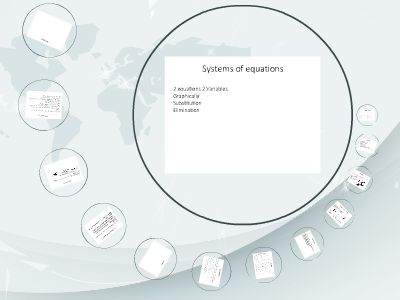Publications: 131 | Followers: 0

## Systems of equations - dsapresents.org

Publish on Category: Birds 0

Systems of equations
2 equations 2 VariablesGraphicallySubstitutionElimination
Solving a System
Getting the ordered pair that makes both equations trueWhere the two lines would intersectYou could have:No solution – meaning they never intersect1 solution they intersect onceInfinite solutions – they are really the same line
Graphically
Solveboth equations for yGraph both of the linesPlot y-intDo slope from y-intConnect with a line3. Find point of intersectionThis works well if lines intersect at an exact point
Example
Solve the following system graphicallyBoth already solvedfor y so graph themThey intersect at(1,-2)Answer should be anOrdered pair
Number of solutions
What would graph look like forNo Solutions 1 Solution Infinite solutions
Equations?
Graphically
This is used a lot in word problemsDetermine which company is better to go with at a certain point and why, intersections tell you this.
Example
Your niece wants to have a birthday party on either McDonalds or Dave and Busters.Given the information below set up a system of equations and graph themMcDonalds: The fixed cost to set up is \$75 and \$5 for each person attending. Write an equation in slope intercept form to represent this.Dave and Busters: The fixed cost to set up is \$50 and \$10 for each person attending. Write an equation in slope intercept form to represent this.
Once you have them graphed answer the following questions.At what number of guest is the cost equal? How do you know?What is the difference in cost for 20 people to go to Dave and Busters vs McDonalds?When is it better to go to Dave and Busters vs McDonalds and when is it better to go to McDonalds vs Dave and Busters?You want to invite 24 people to the part. How much will it cost at each location?You have a \$250 limit on the cost of the party, how many people could you invite to each location?
Substitution
Solve one equation for a variable, look for a variable with a 1 in front of it or an even numberSubstitute the expression you just solved for into the variable of the other equationSolve for the remaining variableTake value of variable and substitute it into the first equationCheck ordered pair in second equationWrite answer as an ordered pair
Example
Number of solutions
How do you determine how many solutions if not graphingNo Solutions – when you solve you get 2 numbers that are not equal to each other1 Solution x and y both equal in number, process worksInfinite solutions – x=x, number equals itself
Solutions
Think back to the equations used for solving graphicallyIf we solve them using substitution
Elimination
Add the equations together to cancel out one of the variablesLine up equations so variables are above one anotherDetermine what to multiply the equations by so when you add them one of the variables will cancelAdd the equations togetherSolve for remaining variableSub in value of variable into original 1stequation and solveCheck answer in second equationWrite answer as an ordered pair
Example

0

Embed

Share

Upload

Make amazing presentation for free
Systems of equations - dsapresents.org